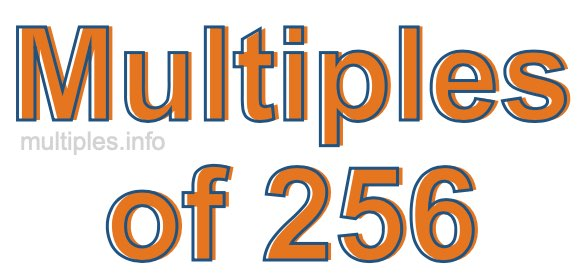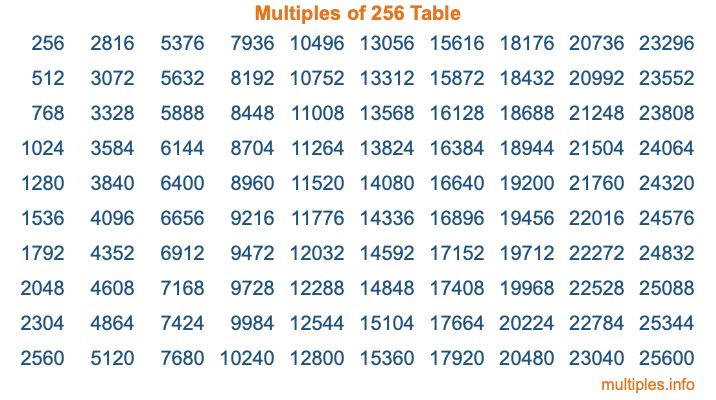Multiples of 256Welcome to the Multiples of 256 page. Here we will first teach you everything you will ever need to know about the multiples of 256, and then give you a study guide summary of everything we taught you to make sure you remember it all. Use this page to look up facts and learn information about the multiples of 256. This page will make you a multiples of two hundred fifty-six expert!

Definition of Multiples of 256
Multiples of 256 are all the numbers that when divided by 256 equal an integer. Each of the multiples of 256 are called a multiple. A multiple of 256 is created by multiplying 256 by an integer.

Therefore, to create a list of multiples of 256, you start with 1 multiplied by 256, then 2 multiplied by 256, then 3 multiplied by 256, and so on for as long as you want. Thus, the list of the first five multiples of 256 is 256, 512, 768, 1024, and 1280. To see a larger list of multiples of 256, see the printable image of Multiples of 256 further down on this page. We also have a category where you can choose any nth multiple of 256.

Multiples of 256 Checker
The Multiples of 256 Checker below checks to see if any number of your choice is a multiple of 256. In other words, it checks to see if there is any number (integer) that when multiplied by 256 will equal your number. To do that, we divide your number by 256. If the the quotient is an integer, then your number is a multiple of 256.

Is  a multiple of 256?

Least Common Multiple of 256 and ...
A Least Common Multiple (LCM) is the lowest multiple that two or more numbers have in common. This is also called the smallest common multiple or lowest common multiple and is useful to know when you are adding our subtracting fractions. Enter one or more numbers below (256 is already entered) to find the LCM.

Check out our LCM Calculator if you need more details about the Least Common Multiple or if you need the LCM for different numbers for adding and subtraction fractions.

nth Multiple of 256
As we stated above, 256 is the first multiple of 256, 512 is the second multiple of 256, 768 is the third multiple of 256, and so on. Enter a number below to find the nth multiple of 256.

th multiple of 256

Multiples of 256 vs Factors of 256
256 is a multiple of 256 and a factor of 256, but that is where the similarities end. All postive multiples of 256 are 256 or greater than 256. All positive factors of 256 are 256 or less than 256.

Below is the beginning list of multiples of 256 and the factors of 256 so you can compare:

Multiples of 256: 256, 512, 768, 1024, 1280, etc.

Factors of 256: 1, 2, 4, 8, 16, 32, 64, 128, 256

As you can see, the multiples of 256 are all the numbers that you can divide by 256 to get a whole number. The factors of 256, on the other hand, are all the whole numbers that you can multiply by another whole number to get 256.

It's also interesting to note that if a number (x) is a factor of 256, then 256 will also be a multiple of that number (x).

Multiples of 256 vs Divisors of 256
The divisors of 256 are all the integers that 256 can be divided by evenly. Below is a list of the divisors of 256.

Divisors of 256: 1, 2, 4, 8, 16, 32, 64, 128, 256

The interesting thing to note here is that if you take any multiple of 256 and divide it by a divisor of 256, you will see that the quotient is an integer.

Multiples of 256 Table
Below is an image of the first 100 multiples of 256 in a table. The table is in chronological order, column by column. The first column has the first ten multiples of 256, the second column has the next ten multiples of 256, and so on.The Multiples of 256 Table is also referred to as the 256 Times Table or Times Table of 256. You are welcome to print out our table for your studies.

Negative Multiples of 256
Although not often discussed or needed in math, it is worth mentioning that you can make a list of negative multiples of 256 by multiplying 256 by -1, then by -2, then by -3, and so on, to get the following list of negative multiples of 256:

-256, -512, -768, -1024, -1280, etc.

Multiples of 256 Summary
Below is a summary of important Multiples of 256 facts that we have discussed on this page. To retain the knowledge on this page, we recommend that you read through the summary and explain to yourself or a study partner why they hold true.

There are an infinite number of multiples of 256.

A multiple of 256 divided by 256 will equal a whole number.

256 divided by a factor of 256 equals a divisor of 256.

The nth multiple of 256 is n times 256.

The largest factor of 256 is equal to the first positive multiple of 256.

256 is a multiple of every factor of 256.

256 is a multiple of 256.

A multiple of 256 divided by a divisor of 256 equals an integer.

256 divided by a divisor of 256 equals a factor of 256.

Any integer times 256 will equal a multiple of 256.

Multiples of a Number
Here you can get the multiples of another number, all with the same attention to detail as we did for multiples of 256 on this page.

Multiples of
Multiples of 257
Did you find our page about multiples of two hundred fifty-six educational? Do you want more knowledge? Check out the multiples of the next number on our list!